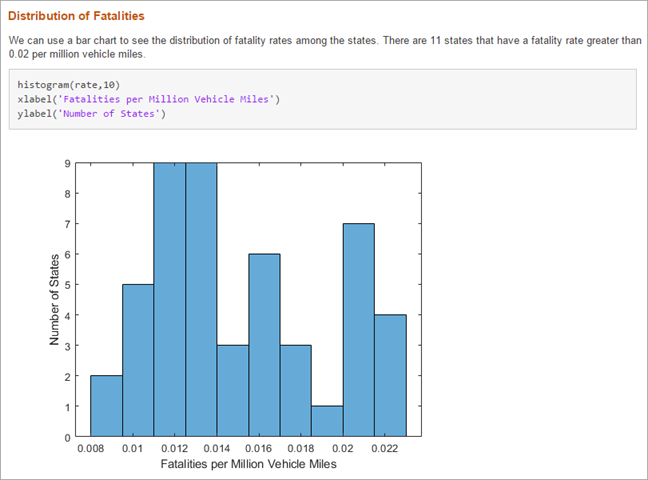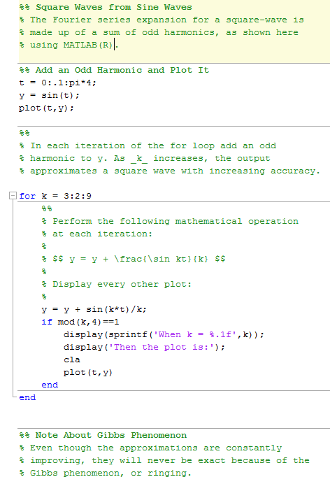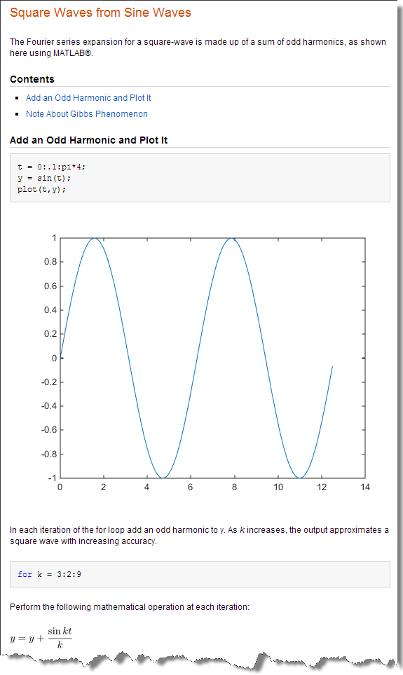## 发布和共享 MATLAB 代码

MATLAB® 提供向他人展示代码的选项。您可以通过创建格式化文档来发布您的 MATLAB 代码文件 (.m)，也可以在实时编辑器中创建和共享实时脚本和实时函数。

### 在实时编辑器中创建和共享实时脚本### 发布 MATLAB 代码文件 (.m)1. 创建一个 MATLAB 脚本或函数。通过在每个部分的开头插入两个百分比符号 (%%) 来将代码划分为多个步骤或节。

2. 通过在文件开头及每节中添加说明性注释来记录代码。

在各节顶部的注释中，您可以添加标记来增强输出的可读性。例如，上表中的代码包括以下标记。

 标题 %% Square Waves from Sine Waves%% Add an Odd Harmonic and Plot It%% Note About Gibbs Phenomenon 斜体格式的变量名称 % As _k_ increases, ... LaTeX 方程 % $$y = y + \frac{sin(k*t)}{k}$$

注意

如果您文件中的文本所包含的字符编码与您的平台编码不同，那么在您保存或发布文件时，MATLAB 会将这些字符显示为乱码。

3. 发布代码。在发布选项卡上，点击

默认情况下，MATLAB 为您的代码创建的每个图形创建一个名为 html 的子文件夹，其中包含一个或多个 HTML 文件。HTML 文件包括代码、格式化注释和输出。您也可以发布为其他格式，例如 PDF 文件或 Microsoft PowerPoint® 演示文稿。有关发布为其他格式的详细信息，请参阅指定输出文件

MATLAB Online™ 中，要允许 MATLAB 在发布时自动打开输出窗口，请在您的 Web 浏览器中启用弹出窗口。